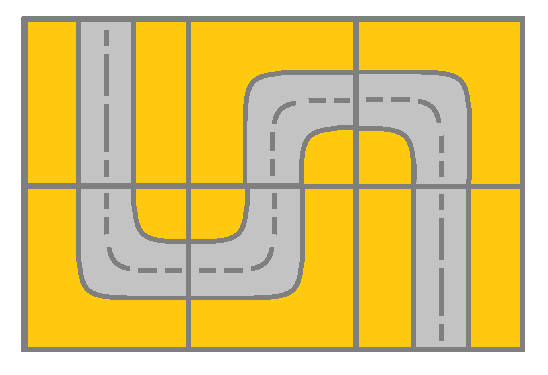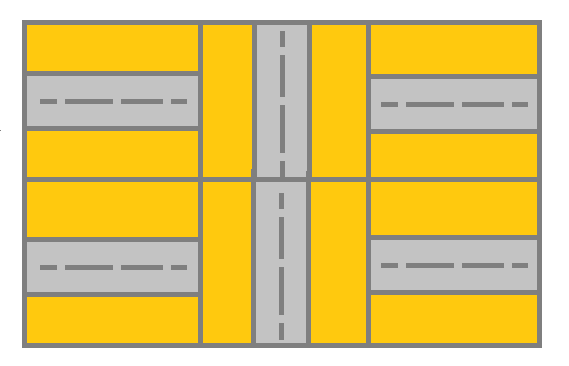GeeksforGeeks App
Open AppBrowser
Continue

# Check if path exists from top-left to bottom-right corner of Grid

Given a grid of dimension M * N where each cell varies from 1 to 6 and each of the values present in the cell represents a path. The task is to check if there exists a path from the top-left corner to the bottom-right corner.  The values present in the cell has the following meaning:

• If the cell value is 1 then it means that there exists a path connecting the left cell and the right cell.
• If the cell value is 2 then it means that there exists a path connecting the upper cell and the lower cell.
• If the cell value is 3 then it means that there exists a path connecting the left cell and the lower cell.
• If the cell value is 4 then it means that there exists a path connecting the right cell and the lower cell.
• If the cell value is 5 then it means that there exists a path connecting the left cell and the upper cell.
• If the cell value is 6 then it means that there exists a path connecting the right cell and the upper cell.

Examples:

Input: grid = [[2, 4, 3], [6, 5, 2]]
Output: True
Explanation:Pictorial Representation of Example 1

As shown you can start at cell (0, 0) and visit all the cells of the grid to reach (m – 1, n – 1).

Input: grid = [[1, 2, 1], [1, 2, 1]]
Output: false
Explanation:Pictorial Representation of Example 2

As you can see that the street at cell (0, 0) is not connected with any street of any other cell and you will get stuck at cell (0, 0).

Approach: To solve the problem follow the below idea:

The problem can be solved by performing Depth-first Search (DFS) on the grid. Check the value present in the current cell and the cell we want to traverse next, and based on that continue the DFS. If the last cell is reached, a path is possible.

Follow the steps to solve the problem:

• Make a 2d matrix (say visited[][]) for tracing the cells that have already been visited.
• Call the recursive function that will check and return the answer.
• Take four variables namely up, down, left, and right representing the four directions in which one can move.
• Based on the current cell value make the respective variable true and then call the recursive function in the respective direction if there exists a path.

Below is the implementation of the above approach:

## C++

 `// C++ code to implement the approach` `#include ``using` `namespace` `std;` `// Function to check the validity of the``// cell index so that it won't go outside``// of the grid``bool` `is_valid(``int` `x, ``int` `y, vector >& grid)``{``    ``if` `(x < 0 || y < 0 || x >= grid.size()``        ``|| y >= grid.size()) {``        ``return` `0;``    ``}``    ``return` `1;``}` `// Recursive function to check if there``// exist a path from the top leftmost``// corner to right bottommost corner x and``// y are coordinates of current cell``void` `dfs(``int` `x, ``int` `y, vector >& visited,``         ``vector >& grid)``{``    ``if` `(visited[x][y])``        ``return``;``    ``visited[x][y] = 1;``    ``int` `up = 0, down = 0, left = 0, right = 0;``    ``if` `(grid[x][y] == 1) {``        ``left = 1;``        ``right = 1;``    ``}``    ``else` `if` `(grid[x][y] == 2) {``        ``up = 1;``        ``down = 1;``    ``}``    ``else` `if` `(grid[x][y] == 3) {``        ``left = 1;``        ``down = 1;``    ``}``    ``else` `if` `(grid[x][y] == 4) {``        ``down = 1;``        ``right = 1;``    ``}``    ``else` `if` `(grid[x][y] == 5) {``        ``up = 1;``        ``left = 1;``    ``}``    ``else` `{``        ``right = 1;``        ``up = 1;``    ``}` `    ``if` `(up) {``        ``int` `new_x = x - 1, new_y = y;``        ``if` `(is_valid(new_x, new_y, grid)``            ``&& (grid[new_x][new_y] == 2``                ``|| grid[new_x][new_y] == 3``                ``|| grid[new_x][new_y] == 4)) {``            ``dfs(new_x, new_y, visited, grid);``        ``}``    ``}``    ``if` `(down) {``        ``int` `new_x = x + 1, new_y = y;``        ``if` `(is_valid(new_x, new_y, grid)``            ``&& (grid[new_x][new_y] == 2``                ``|| grid[new_x][new_y] == 5``                ``|| grid[new_x][new_y] == 6)) {``            ``dfs(new_x, new_y, visited, grid);``        ``}``    ``}``    ``if` `(left) {``        ``int` `new_x = x, new_y = y - 1;``        ``if` `(is_valid(new_x, new_y, grid)``            ``&& (grid[new_x][new_y] == 1``                ``|| grid[new_x][new_y] == 4``                ``|| grid[new_x][new_y] == 6)) {``            ``dfs(new_x, new_y, visited, grid);``        ``}``    ``}``    ``if` `(right) {``        ``int` `new_x = x, new_y = y + 1;``        ``if` `(is_valid(new_x, new_y, grid)``            ``&& (grid[new_x][new_y] == 1``                ``|| grid[new_x][new_y] == 3``                ``|| grid[new_x][new_y] == 5)) {``            ``dfs(new_x, new_y, visited, grid);``        ``}``    ``}``}` `// Driver code``int` `main()``{``    ``vector > grid``        ``= { { 2, 4, 3 }, { 6, 5, 2 } };``    ``int` `N = grid.size(), M = grid.size();``    ``vector > visited(N, vector<``bool``>(M, 0));` `    ``// Function Call``    ``dfs(0, 0, visited, grid);``    ``if` `(visited[N - 1][M - 1])``        ``cout << ``"Possible\n"``;``    ``else``        ``cout << ``"Impossible\n"``;` `    ``return` `0;``}`

## Java

 `// Java code to implement the approach` `import` `java.io.*;` `class` `GFG {` `    ``// Function to check the validity of the``    ``// cell index so that it won't go outside``    ``// of the grid``    ``static` `boolean` `is_valid(``int` `x, ``int` `y, ``int``[][] grid)``    ``{``        ``if` `(x < ``0` `|| y < ``0` `|| x >= grid.length``            ``|| y >= grid[``0``].length) {``            ``return` `false``;``        ``}``        ``return` `true``;``    ``}` `    ``// Recursive function to check if there``    ``// exist a path from the top leftmost``    ``// corner to right bottommost corner x and``    ``// y are coordinates of current cell``    ``static` `void` `dfs(``int` `x, ``int` `y, ``boolean``[][] visited,``                    ``int``[][] grid)``    ``{``        ``if` `(visited[x][y]) {``            ``return``;``        ``}``        ``visited[x][y] = ``true``;``        ``int` `up = ``0``, down = ``0``, left = ``0``, right = ``0``;``        ``if` `(grid[x][y] == ``1``) {``            ``left = ``1``;``            ``right = ``1``;``        ``}``        ``else` `if` `(grid[x][y] == ``2``) {``            ``up = ``1``;``            ``down = ``1``;``        ``}``        ``else` `if` `(grid[x][y] == ``3``) {``            ``left = ``1``;``            ``down = ``1``;``        ``}``        ``else` `if` `(grid[x][y] == ``4``) {``            ``down = ``1``;``            ``right = ``1``;``        ``}``        ``else` `if` `(grid[x][y] == ``5``) {``            ``up = ``1``;``            ``left = ``1``;``        ``}``        ``else` `{``            ``right = ``1``;``            ``up = ``1``;``        ``}``        ``if` `(up != ``0``) {``            ``int` `new_x = x - ``1``, new_y = y;``            ``if` `(is_valid(new_x, new_y, grid)``                ``&& (grid[new_x][new_y] == ``2``                    ``|| grid[new_x][new_y] == ``3``                    ``|| grid[new_x][new_y] == ``4``)) {``                ``dfs(new_x, new_y, visited, grid);``            ``}``        ``}``        ``if` `(down != ``0``) {``            ``int` `new_x = x + ``1``, new_y = y;``            ``if` `(is_valid(new_x, new_y, grid)``                ``&& (grid[new_x][new_y] == ``2``                    ``|| grid[new_x][new_y] == ``5``                    ``|| grid[new_x][new_y] == ``6``)) {``                ``dfs(new_x, new_y, visited, grid);``            ``}``        ``}``        ``if` `(left != ``0``) {``            ``int` `new_x = x, new_y = y - ``1``;``            ``if` `(is_valid(new_x, new_y, grid)``                ``&& (grid[new_x][new_y] == ``1``                    ``|| grid[new_x][new_y] == ``4``                    ``|| grid[new_x][new_y] == ``6``)) {``                ``dfs(new_x, new_y, visited, grid);``            ``}``        ``}``        ``if` `(right != ``0``) {``            ``int` `new_x = x, new_y = y + ``1``;``            ``if` `(is_valid(new_x, new_y, grid)``                ``&& (grid[new_x][new_y] == ``1``                    ``|| grid[new_x][new_y] == ``3``                    ``|| grid[new_x][new_y] == ``5``)) {``                ``dfs(new_x, new_y, visited, grid);``            ``}``        ``}``    ``}` `    ``public` `static` `void` `main(String[] args)``    ``{``        ``int``[][] grid = { { ``2``, ``4``, ``3` `}, { ``6``, ``5``, ``2` `} };``        ``int` `N = grid.length;``        ``int` `M = grid[``0``].length;``        ``boolean``[][] visited = ``new` `boolean``[N][M];` `        ``// Function call``        ``dfs(``0``, ``0``, visited, grid);``        ``if` `(visited[N - ``1``][M - ``1``]) {``            ``System.out.println(``"Possible"``);``        ``}``        ``else` `{``            ``System.out.println(``"Impossible"``);``        ``}``    ``}``}` `// This code is contributed by lokeshmvs21.`

## Python3

 `# Python code to implement the approach` `# Function to check the validity of the``# cell index so that it won't go outside``# of the grid``def` `is_valid(x, y, grid):``    ``if` `x < ``0` `or` `y < ``0` `or` `x >``=` `len``(grid) ``or` `y >``=` `len``(grid[``0``]):``        ``return` `False``    ``return` `True` `# Recursive function to check if there``# exist a path from the top leftmost``# corner to right bottommost corner x and``# y are coordinates of current cell``def` `dfs(x, y, visited, grid):``    ``if` `visited[x][y]:``        ``return``    ``visited[x][y] ``=` `True``    ``up ``=` `0``    ``down ``=` `0``    ``left ``=` `0``    ``right ``=` `0``    ``if` `grid[x][y] ``=``=` `1``:``        ``left ``=` `1``        ``right ``=` `1``    ``elif` `grid[x][y] ``=``=` `2``:``        ``up ``=` `1``        ``down ``=` `1``    ``elif` `grid[x][y] ``=``=` `3``:``        ``left ``=` `1``        ``down ``=` `1``    ``elif` `grid[x][y] ``=``=` `4``:``        ``down ``=` `1``        ``right ``=` `1``    ``elif` `grid[x][y] ``=``=` `5``:``        ``up ``=` `1``        ``left ``=` `1``    ``else``:``        ``right ``=` `1``        ``up ``=` `1` `    ``if` `up:``        ``new_x ``=` `x ``-` `1``        ``new_y ``=` `y``        ``if` `is_valid(new_x, new_y, grid) ``and` `(grid[new_x][new_y] ``=``=` `2` `or` `grid[new_x][new_y] ``=``=` `3` `or` `grid[new_x][new_y] ``=``=` `4``):``            ``dfs(new_x, new_y, visited, grid)``    ``if` `down:``        ``new_x ``=` `x ``+` `1``        ``new_y ``=` `y``        ``if` `is_valid(new_x, new_y, grid) ``and` `(grid[new_x][new_y] ``=``=` `2` `or` `grid[new_x][new_y] ``=``=` `5` `or` `grid[new_x][new_y] ``=``=` `6``):``            ``dfs(new_x, new_y, visited, grid)``    ``if` `left:``        ``new_x ``=` `x``        ``new_y ``=` `y ``-` `1``        ``if` `is_valid(new_x, new_y, grid) ``and` `(grid[new_x][new_y] ``=``=` `1` `or` `grid[new_x][new_y] ``=``=` `4` `or` `grid[new_x][new_y] ``=``=` `6``):``            ``dfs(new_x, new_y, visited, grid)``    ``if` `right:``        ``new_x ``=` `x``        ``new_y ``=` `y ``+` `1``        ``if` `is_valid(new_x, new_y, grid) ``and` `(grid[new_x][new_y] ``=``=` `1` `or` `grid[new_x][new_y] ``=``=` `3` `or` `grid[new_x][new_y] ``=``=` `5``):``            ``dfs(new_x, new_y, visited, grid)`  `# Driver code``grid ``=` `[[``2``, ``4``, ``3``], [``6``, ``5``, ``2``]]``N ``=` `len``(grid)``M ``=` `len``(grid[``0``])``visited ``=` `[[``False` `for` `j ``in` `range``(M)] ``for` `i ``in` `range``(N)]` `# Function call``dfs(``0``, ``0``, visited, grid)``if``(visited[N``-``1``][M``-``1``]):``    ``print``(``"Possible"``)``else``:``    ``print``(``"Impossible"``)` `# This code is contributed by lokesh.`

## C#

 `// C# code to implement the approach``using` `System;` `class` `GFG {` `    ``// Function to check the validity of the``    ``// cell index so that it won't go outside``    ``// of the grid``    ``static` `bool` `is_valid(``int` `x, ``int` `y, ``int``[,] grid)``    ``{``        ``if` `(x < 0 || y < 0 || x >= grid.GetLength(0)``            ``|| y >= grid.GetLength(1)) {``            ``return` `false``;``        ``}``        ``return` `true``;``    ``}` `    ``// Recursive function to check if there``    ``// exist a path from the top leftmost``    ``// corner to right bottommost corner x and``    ``// y are coordinates of current cell``    ``static` `void` `dfs(``int` `x, ``int` `y, ``bool``[,] visited,``                    ``int``[,] grid)``    ``{``        ``if` `(visited[x,y]) {``            ``return``;``        ``}``        ``visited[x,y] = ``true``;``        ``int` `up = 0, down = 0, left = 0, right = 0;``        ``if` `(grid[x,y] == 1) {``            ``left = 1;``            ``right = 1;``        ``}``        ``else` `if` `(grid[x,y] == 2) {``            ``up = 1;``            ``down = 1;``        ``}``        ``else` `if` `(grid[x,y] == 3) {``            ``left = 1;``            ``down = 1;``        ``}``        ``else` `if` `(grid[x,y] == 4) {``            ``down = 1;``            ``right = 1;``        ``}``        ``else` `if` `(grid[x,y] == 5) {``            ``up = 1;``            ``left = 1;``        ``}``        ``else` `{``            ``right = 1;``            ``up = 1;``        ``}``        ``if` `(up != 0) {``            ``int` `new_x = x - 1, new_y = y;``            ``if` `(is_valid(new_x, new_y, grid)``                ``&& (grid[new_x,new_y] == 2``                    ``|| grid[new_x,new_y] == 3``                    ``|| grid[new_x,new_y] == 4)) {``                ``dfs(new_x, new_y, visited, grid);``            ``}``        ``}``        ``if` `(down != 0) {``            ``int` `new_x = x + 1, new_y = y;``            ``if` `(is_valid(new_x, new_y, grid)``                ``&& (grid[new_x,new_y] == 2``                    ``|| grid[new_x,new_y] == 5``                    ``|| grid[new_x,new_y] == 6)) {``                ``dfs(new_x, new_y, visited, grid);``            ``}``        ``}``        ``if` `(left != 0) {``            ``int` `new_x = x, new_y = y - 1;``            ``if` `(is_valid(new_x, new_y, grid)``                ``&& (grid[new_x,new_y] == 1``                    ``|| grid[new_x,new_y] == 4``                    ``|| grid[new_x,new_y] == 6)) {``                ``dfs(new_x, new_y, visited, grid);``            ``}``        ``}``        ``if` `(right != 0) {``            ``int` `new_x = x, new_y = y + 1;``            ``if` `(is_valid(new_x, new_y, grid)``                ``&& (grid[new_x,new_y] == 1``                    ``|| grid[new_x,new_y] == 3``                    ``|| grid[new_x,new_y] == 5)) {``                ``dfs(new_x, new_y, visited, grid);``            ``}``        ``}``    ``}` `    ``public` `static` `void` `Main()``    ``{``        ``int``[,] grid = { { 2, 4, 3 }, { 6, 5, 2 } };``        ``int` `N = grid.GetLength(0);``        ``int` `M = grid.GetLength(1);``        ``bool``[,] visited = ``new` `bool``[N,M];` `        ``// Function call``        ``dfs(0, 0, visited, grid);``        ``if` `(visited[N - 1,M - 1]) {``            ``Console.WriteLine(``"Possible"``);``        ``}``        ``else` `{``            ``Console.WriteLine(``"Impossible"``);``        ``}``    ``}``}` `// This code is contributed by Pushpesh Raj.`

## Javascript

 `// Javascript code to implement the approach` `// Function to check the validity of the``// cell index so that it won't go outside``// of the grid``function` `is_valid( x,  y, grid)``{``    ``if` `(x < 0 || y < 0 || x >= grid.length``        ``|| y >= grid.length) {``        ``return` `false``;``    ``}``    ``return` `true``;``}` `// Recursive function to check if there``// exist a path from the top leftmost``// corner to right bottommost corner x and``// y are coordinates of current cell``function` `dfs(x,  y, visited, grid)``{``    ``if` `(visited[x][y] == ``true``)``        ``return``;``    ``visited[x][y] = ``true``;``    ``let up = 0, down = 0, left = 0, right = 0;``    ``if` `(grid[x][y] == 1) {``        ``left = 1;``        ``right = 1;``    ``}``    ``else` `if` `(grid[x][y] == 2) {``        ``up = 1;``        ``down = 1;``    ``}``    ``else` `if` `(grid[x][y] == 3) {``        ``left = 1;``        ``down = 1;``    ``}``    ``else` `if` `(grid[x][y] == 4) {``        ``down = 1;``        ``right = 1;``    ``}``    ``else` `if` `(grid[x][y] == 5) {``        ``up = 1;``        ``left = 1;``    ``}``    ``else` `{``        ``right = 1;``        ``up = 1;``    ``}` `    ``if` `(up != 0) {``        ``let new_x = x - 1, new_y = y;``        ``if` `(is_valid(new_x, new_y, grid)==``true``            ``&& (grid[new_x][new_y] == 2``                ``|| grid[new_x][new_y] == 3``                ``|| grid[new_x][new_y] == 4)) {``            ``dfs(new_x, new_y, visited, grid);``        ``}``    ``}``    ``if` `(down != 0) {``        ``let new_x = x + 1, new_y = y;``        ``if` `(is_valid(new_x, new_y, grid)==``true``            ``&& (grid[new_x][new_y] == 2``                ``|| grid[new_x][new_y] == 5``                ``|| grid[new_x][new_y] == 6)) {``            ``dfs(new_x, new_y, visited, grid);``        ``}``    ``}``    ``if` `(left != 0) {``        ``let new_x = x, new_y = y - 1;``        ``if` `(is_valid(new_x, new_y, grid)==``true``            ``&& (grid[new_x][new_y] == 1``                ``|| grid[new_x][new_y] == 4``                ``|| grid[new_x][new_y] == 6)) {``            ``dfs(new_x, new_y, visited, grid);``        ``}``    ``}``    ``if` `(right!=0) {``        ``let new_x = x, new_y = y + 1;``        ``if` `(is_valid(new_x, new_y, grid)==``true``            ``&& (grid[new_x][new_y] == 1``                ``|| grid[new_x][new_y] == 3``                ``|| grid[new_x][new_y] == 5)) {``            ``dfs(new_x, new_y, visited, grid);``        ``}``    ``}``}` `// Driver code``    ``let grid``        ``= [[ 2, 4, 3 ], [ 6, 5, 2 ]];``    ``let N = grid.length, M = grid.length;``    ``let visited = ``new` `Array(N);``    ``for``(let i = 0; i < N; i++)``    ``{``        ``visited[i]= ``new` `Array(M);``        ``for``(let j = 0; j < M; j++)``            ``visited[i][j] = ``false``;``    ``}``    `  `    ``// Function Call``    ``dfs(0, 0, visited, grid);``    ``if` `(visited[N - 1][M - 1] == ``true``)``        ``console.log( ``"Possible"``);``    ``else``       ``console.log(``"Impossible"``);` `// This code is contributed by garg28harsh.`

Output

`Possible`

Time complexity: O(M * N) because we have to call the recursive function for every cell.
Auxiliary Space: O(M * N)

Related Articles:

My Personal Notes arrow_drop_up